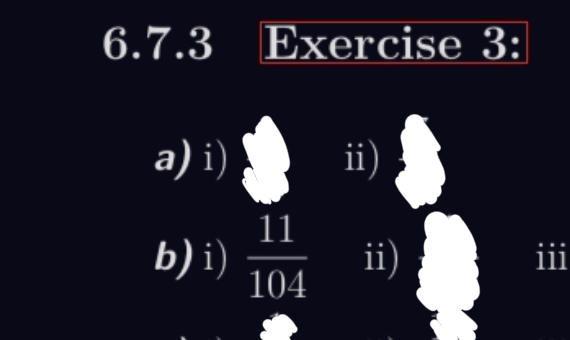# Math Probability Question

Hi I need lots of help with probabilityAccording to the answer sheet, it seems like the integer of the denominator should be divided by 3; but I don’t get the idea how.

Hi

that’s strange my answer is 11/52 and i divided the denominator by 6 not 3
could someone find what is wrong with my reasoning?

possible tree diagrams:
-p-g-b
-p-b-g
-g-p-b
-g-b-p
-b-g-p
-b-p-g

P (pgb) = (5 * 10 * 11)/(26 * 25 * 24) = 11/312
Ptotal = 6 * 11/312 = 11/52

1 Like